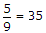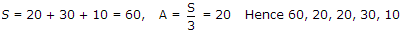# Electronics and Communication Engineering - Microprocessors

### Exercise :: Microprocessors - Section 3

11.

Consider the following statements about expressing real constants in exponential form in C

1. The mantissa and exponent are separated either by e or E.
2. The mantissa and exponent may have positive or negative sign.
3. Default sign of mantissa is positive.
4. Default sign of exponent is negative.
Which of the above statements are wrong?

 A. 1 only B. 1 and 4 only C. 1, 3 and 4 only D. 4 only

Explanation:

E is not allowed and default sign of exponent is positive.

12.

If F = 95.0 what will be the result of following FORTRAN 77 program? `REAL F , CREAD * , FC = (5. / 9.) * (F - 32.)`

 A. 35 B. 35 C. 25 D. 25

Explanation:

(95 - 32)and decimal must be added.

13.

Consider the following library functions

1. `EXP (i.e., ex)`
2. `ARCTAN (i.e. tan-1 x)`
3. `SQRT (i.e., x)`
4. `AES (i.e. |x|)`

Which of the above are allowed in Pascal?

 A. All B. 1 and 3 only C. 1, 3, 4 only D. 1, 2 and 3 only

Explanation:

All are allowed in Pascal.

14.

Consider the following program in Basic

`10 READ X, Y, Z15 DATA 20, 30, 1020 S = X + Y + Z30 A = S/335 PRINT S, A, X, Y, Z40 END`

The output will be

 A. 60, 20, 20, 30, 10 B. 10, 30, 20, 20, 60 C. 60, 20, 30, 10, 20 D. 60, 30, 10, 20, 20

Explanation:.

15.

Let the initial contents of `ACC and CY` in 8085 be

1. `CY = 1`
2. `ACC = 10000001`
After the instruction `RAL` is executed twice the contents of `CY and ACC` respectively will be

 A. 1 and 00000011 B. 0 and 00000111 C. 1 and 00000111 D. 0 and 00000011